# Design S-Band Monopulse Tracking Radar Antenna

This example shows how to implement a monopulse operation by integrating a 2-by-4 microstrip patch array and a rat-race coupler.

You design the system for an operating frequency of 3 GHz and compare the simulated and measured results. The antenna attains a maximum gain of 15.3 dB at the sum port.

This diagram shows the top view of the antenna.### Create Antenna Array Geometry

Create a rat-race coupler that resonates at 3 GHz using the `couplerRatrace` object.

```cup = couplerRatrace; d = cup.Substrate; cup.PortLineLength = 18.6e-3; cup.PortLineWidth = 0.0050; cup.CouplerLineWidth = 0.0030; cup.Circumference = 0.111;```

Use the `pcbComponent` object to form a PCB stack of the rat-race couplers such that the ground plane can be extended to accommodate the patch antenna array.

```RR = pcbComponent(cup); RR.BoardShape = antenna.Rectangle(Center=[0 0.02],Length=0.4,Width=0.22); RR.Layers{3} = RR.BoardShape; ground = RR.BoardShape;```

Arrange the rat-race coupler at the center of the patch antennas such that the distances between the patch antennas on both sides are the same. To modify the rat-race coupler for this design, use the `antenna.Rectangle` (Antenna Toolbox) object and Boolean addition and subtraction operations. Visualize the structure using the `show` (Antenna Toolbox) function.

```top = RR.Layers{1}; cut1 = antenna.Rectangle(Center=[34.4e-3 0e-3],Length=3e-3,Width=5e-3); cut2 = antenna.Rectangle(Center=[-34.4e-3 0e-3],Length=3e-3,Width=5e-3); top = top - cut1 - cut2; top = rotateZ(top,-30); cutfifine1 = antenna.Rectangle(Center=[0 35.9e-3],Length=7e-3,Width=6e-3); top = top - cutfifine1; top = rotateZ(top,-30); cutfifine2 = antenna.Rectangle(Center=[35.9e-3 0],Length=6e-3,Width=7e-3); top = top - cutfifine2; top = rotateZ(top,30); top = translate(top,[0,28e-3,0]); figure(Name="Rat-race Coupler") show(top)```### Create patch antenna

Create the patch antenna array using the `patchMicrostripInsetfed` (Antenna Toolbox) object. Assign property values such that the antenna resonates at 3 GHz.

```ant = patchMicrostripInsetfed; ant.Length = 33.6309e-3; ant.Width = 40.1597e-3; ant.Height = 1.6e-3; ant.Substrate = d; ant.NotchLength = 7e-3; ant.GroundPlaneLength = 0.0600; ant.StripLineWidth = 5e-3; ant.NotchWidth = 5.577e-3;```

### Create antenna array

Convert the antenna to a PCB stack using the `pcbStack` (Antenna Toolbox) object and copy the designed antenna element to create eight identical elements. Position the patch antennas on both the sides of the rat-race coupler to create a 2-by-4 array using the `translate` (Antenna Toolbox) function. Visualize the antennas using the `show` function.

```array = pcbStack(ant); a1 = array.Layers{1}; a1 = rotateZ(a1,90); a2 = copy(a1); a3 = copy(a1); a4 = copy(a1); ant1 = translate(a1,[-125.5202e-3 69e-3,0]); ant2 = translate(a2,[-125.5202e-3 -0.008650,0]); ant3 = translate(a3,[-44.3591e-3 69e-3,0]); ant4 = translate(a4,[-44.3591e-3 -0.008650,0]); antArrayleft = ant1 + ant3 + ant2 + ant4; antArrayright = copy(antArrayleft); antArrayright = mirrorY(antArrayright); antArray = antArrayleft + antArrayright; figure(Name="Microstrip Patch Array") show(antArray)```Create a part of the power divider network using the `antenna.Rectangle` (Antenna Toolbox) object. Create the power divider using the `traceTee` object. Create four power dividers with the same dimensions using the `copy` and `mirrorX` (Antenna Toolbox) functions.

```inline1 = antenna.Rectangle(Center=[-54.29e-3 0.0405],Length=15e-3,Width=2.9e-3); inline2= antenna.Rectangle(Center=[-115.6e-3 0.0405],Length=15e-3,Width=2.9e-3); inline3 = antenna.Rectangle(Center=[-54.29e-3 -37.1475e-3],Length=15e-3,Width=2.9e-3); inline4 = antenna.Rectangle(Center=[-115.6e-3 -37.1475e-3],Length=15e-3,Width=2.9e-3); tee = traceTee; tee.Length = [46.3746e-3 18e-3]; tee.Width = [1.492e-3 5e-3]; tee1 = copy(tee); tee2 = copy(tee); tee3 = copy(tee); tee.ReferencePoint = [84.92e-3 0.0405]; tee2 = mirrorX(tee2); tee3 = mirrorX(tee3); tee1.ReferencePoint = [-84.92e-3 0.0405]; tee2.ReferencePoint = [84.92e-3 37.1475e-3]; tee3.ReferencePoint = [-84.92e-3 37.1475e-3]; teecut = antenna.Circle(Center=[12e-3 12e-3],Radius=3e-3,NumPoints=3); ytee = traceTee; ytee.Length = [45.3746e-3 9.12456e-3]; ytee.Width = [1.492e-3 5e-3]; y1tee = copy(ytee); ytee.ReferencePoint = [-1.675e-3 84.92e-3]; ytee = rotateZ(ytee,-90); y1tee.ReferencePoint = [1.675e-3 84.92e-3]; y1tee = rotateZ(y1tee,90); fifine = antenna.Rectangle(Center=[-73.585e-3 9.175e-3],Length=5e-3,Width=20e-3); fifine1 = antenna.Rectangle(Center=[-52.57e-3 20.105e-3],Length=47e-3,Width=5e-3); fifine2 = antenna.Rectangle(Center=[-27.7e-3 32.6e-3],Length=5e-3,Width=30e-3); curve = antenna.Polygon(Vertices=[-84.085e-3 -0.825e-3 0; -79.085e-3 -0.825e-3 0; ... -79.085e-3 4.175e-3 0]); curve = translate(curve,[8e-3,0,0]); curve1 = antenna.Polygon(Vertices=[-84.085e-3 22.705e-3 0; -84.085e-3 17.605e-3 0; ... -79.085e-3 22.705e-3 0]); curve1 = translate(curve1,[8e-3 0 0]); curve2 = antenna.Polygon(Vertices=[-33.2e-3 17.6e-3 0; -33.2e-3 22.605e-3 0; ... -38.4e-3 17.6e-3 0]); curve2=translate(curve2,[8e-3 0 0]); sqcut = antenna.Rectangle(Center=[-26.7e-3 47.518e-3],Length=7e-3,Width=2.1640e-3); sqcut2 = copy(sqcut); sqcut2 = mirrorY(sqcut2); RRline = fifine + fifine1 + fifine2 - curve - curve1 - curve2; feedLeft = tee1 + tee3 + inline1 + inline2 + inline3 + inline4 + y1tee + RRline; feedRight = copy(feedLeft); feedRight = mirrorY(feedRight); totaltop = top + feedLeft + feedRight + antArray - sqcut - sqcut2;```

### Create PCB antenna from geometry

Create a stack of antenna layers using the `pcbStack` object and assign the shape created in the previous section to the top layer of the stack. This step completes the patch antenna design.

```rrMicrostrip = pcbStack; rrMicrostrip.Layers{1} = totaltop; rrMicrostrip.BoardThickness = 0.0016; rrMicrostrip.BoardShape = antenna.Rectangle(Center=[0 0.02],Length=0.4,Width=0.22); rrMicrostrip.Layers = {totaltop d ground}; feedloc = [[0 58e-3 3 1]; [26e-3 13e-3 3 1]]; rrMicrostrip.FeedLocations = feedloc; rrMicrostrip.FeedDiameter = 0.005/8; rrMicrostrip.FeedViaModel = "strip"; rrMicrostrip.FeedPhase = 0; show(rrMicrostrip)```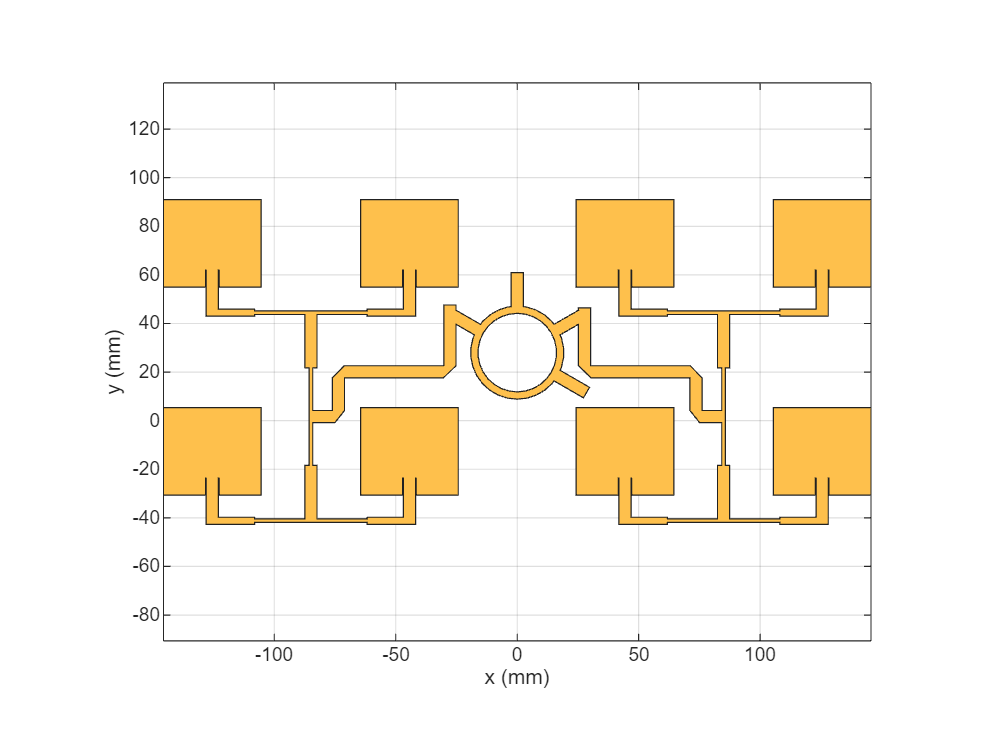### Analyze the Antenna

Set the `FeedVoltage` property of the `pcbStack` object to `[1 0]` to feed a single port amongst two. Use this value to get the sum port characteristics. To get the difference port characteristics, set the `FeedVoltage` property to `[0 1]`.

`rrMicrostrip.FeedVoltage = [1 0];`

Manually mesh the array using the `mesh` (Antenna Toolbox) function. For a finer mesh, set the `MaxEdgeLength` property to one eighth of the wavelength.

```plotfrequency = 3.3*1e9; lambda = 3e8/plotfrequency; mesh(rrMicrostrip,MaxEdgeLength=lambda/8);```### Calculate S`-`parameters

Calculate the memory required to solve the structure using the `memoryEstimate` function.

`memEst = memoryEstimate(rrMicrostrip,3e9)`
```memEst = '2.8 GB' ```

Calculate the S-parameters using the `sparameters` (Antenna Toolbox) function. To save simulation time, use the results in the `RRmicrostripdata` MAT file. To compute the S-parameters without using the MAT file, set the `plotSparameters` variable to true.

```frequencyRange = (2.5:0.1:3.5)*1e9; calculateSparameters = false; if calculateSparameters s = sparameters(rrMicrostrip,frequencyRange); rfplot(s) end```

Load the `sBandmonopulse` MAT file, whch contains the simulated and measured data stored in the variables. Use `S11_simulated` variable to plot the S-parameters.

```load sBandmonopulse.mat figure(Name="S-parameters") plot(simufreq,S11_simulated,"r",LineWidth=2) hold on plot(simufreq,S12_simulated,"b",LineWidth=2) hold off legend("S11","S12") xlabel("Frequency (MHz)") ylabel("Reflection Coefficient") ```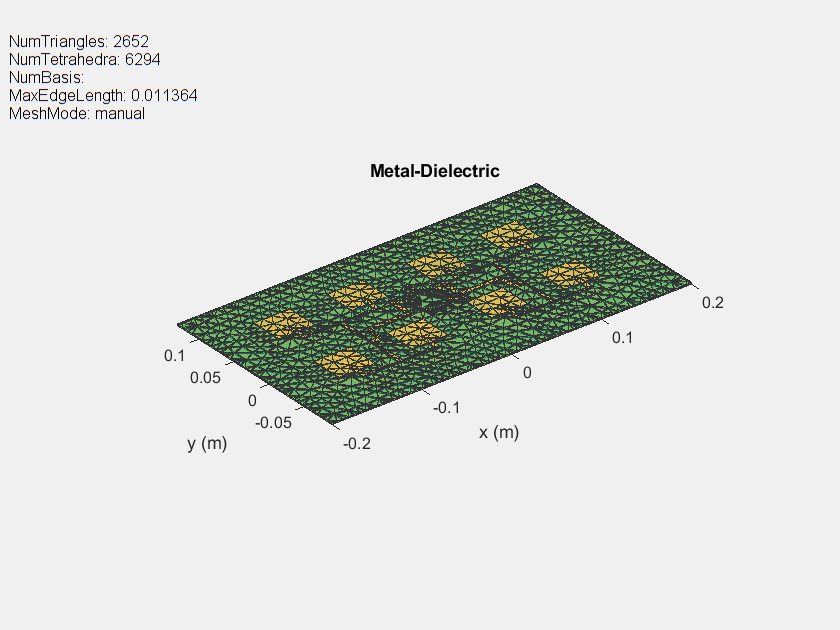Plot the 3-D radiation pattern at 3.3 GHz using the `pattern` (Antenna Toolbox) function.

```figure pattern(rrMicrostrip,3.3e9)```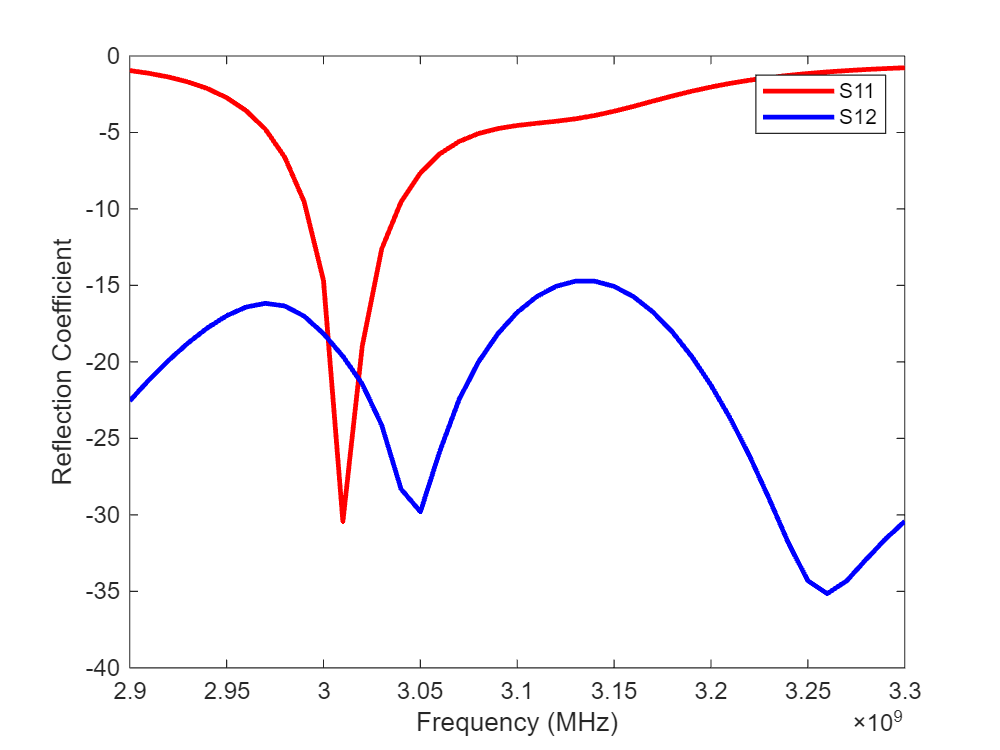Plot the 2-D radiation pattern at an elevation angle of 0 degrees using the `pattern` function`.`

```figure pattern(rrMicrostrip,plotfrequency,0,0:1:360)```The designed array antenna is fabricated as shown below and it is tested for the reflection coefficient and the radiation pattern at the sum and difference ports. Reflection coefficient of fabricated antenna array is measured using a Network Analyzer. Radiation pattern measurements are performed in an anechoic chamber.

This figure shows the top view of the fabricated antenna.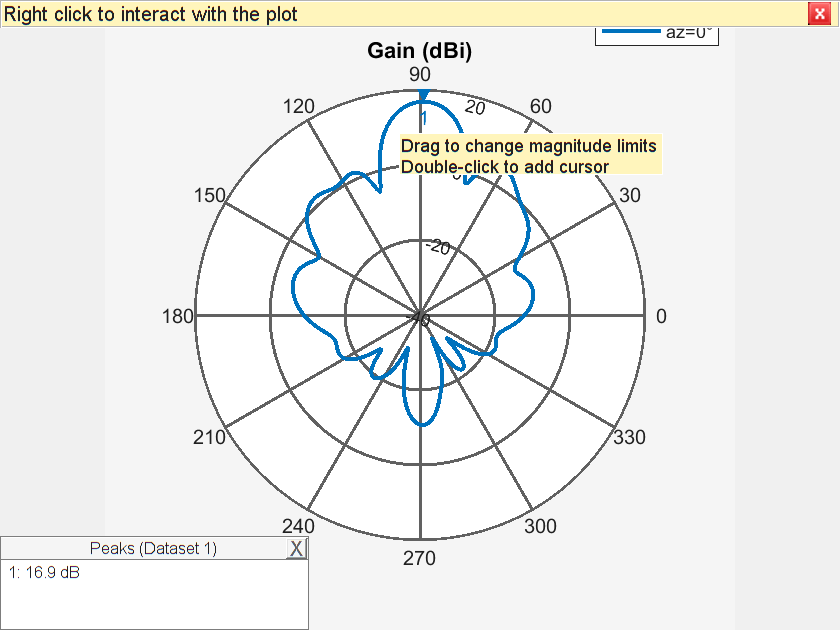### Compare Simulation Results with Measured Results

Plot the measured reflection coefficient.

``` plot(measfreq,S11_measured',"b",LineWidth=2,DisplayName="Measured Reflection Coefficient"); xlabel("Frequency (MHz)"); ylabel("Reflection Coefficient"); ```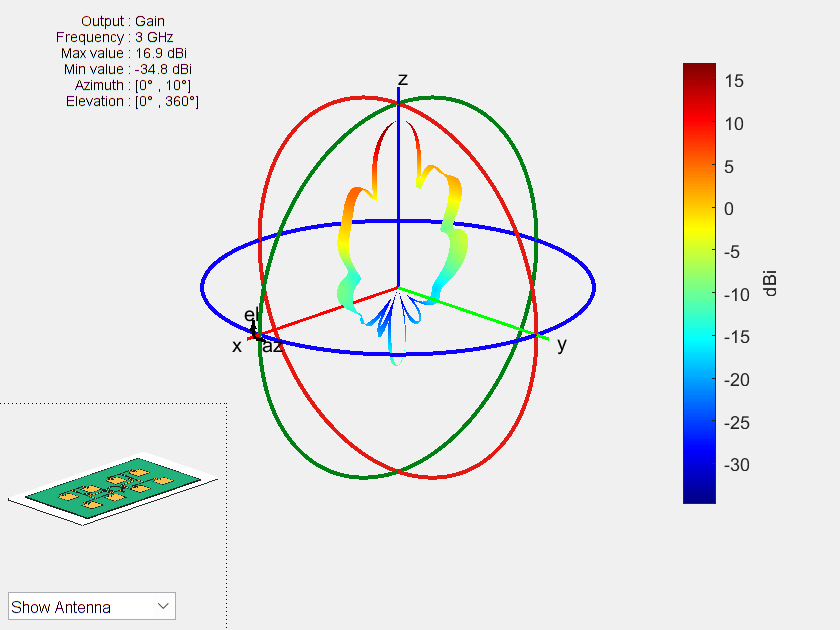Plot the measured sum radiation pattern.

``` plot(-180:10:180,sumpattern_measured',"b",LineWidth=2,DisplayName="Measured Sum Radiation Pattern"); xlabel("Theta (degree)"); ylabel("Normalized Gain (dB)"); ```Plot the measured diffrence radiation pattern.

```plot(-44:2:46,diffpattern_measured,"r",LineWidth=2,DisplayName="Measured Difference Radiation Pattern"); xlabel("Theta(degree)"); ylabel("Normalized Gain (dB)");```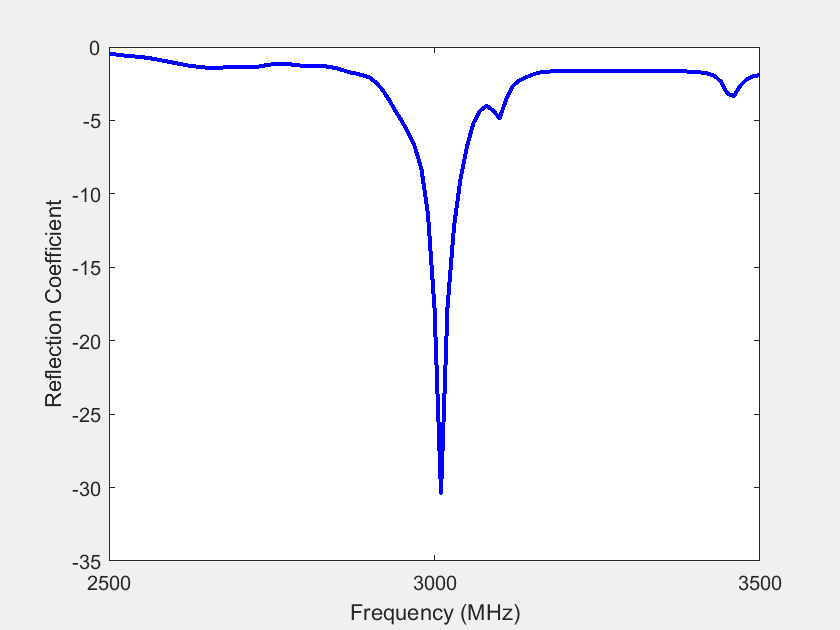### Conclusion

The designed antenna has a maximum sum port gain of 15.3 dB and null depth of 20 dB at the difference port. You confirm the efficiency of the antenna array by plotting the measured and simulated characteristics. The measured and simulated results agree well. The designed array antenna is a promising candidate for monopulse tracking radar in the S-band.

### Reference

 Kumar, Hemant, and Girish Kumar. “Microstrip Antenna Array with Ratrace Comparator at X-Band for Monopulse Tracking Radar.” In 2016 IEEE International Symposium on Antennas and Propagation (APSURSI), 513–14. Fajardo, PR, USA: IEEE, 2016. https://doi.org/10.1109/APS.2016.7695965